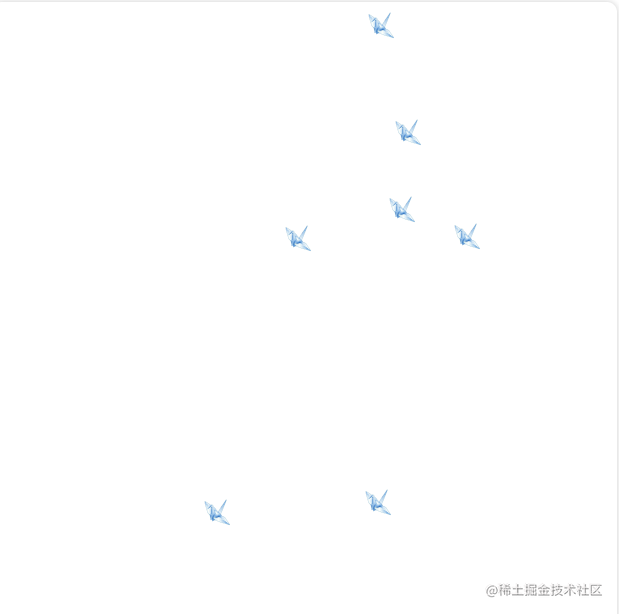# 我用Vue2实现了QQ空间的漂浮千纸鹤效果``````initCranePosition() {
return {
x: (Math.random() * 100 + 200).toFixed(2),
y: 0
};
},
genSpeed() {
return {
vx: (Math.random() * 2 - 1).toFixed(2),
vy: (Math.random() * 5).toFixed(2) + 5,
g: -Math.random().toFixed(2)
};
}

``````genCrane() {
if (this.craneList.length) {
let list = [...this.craneList].filter((item) => item.x > 0 && item.x < 500 && item.y < 500);
this.craneList = list.map((item) => {
item.x = Number(item.x) + Number(item.vx);
item.y = Number(item.y) + Number(item.vy);
if (item.vy > 2) item.vy = Number(item.vy) + Number(item.g);
return item;
});
}
if (this.craneList.length < this.maxLenth) {
const position = this.initCranePosition();
const speed = this.genSpeed();
this.craneList.push({
...speed,
...position
});
}
this.timer = window.requestAnimationFrame(this.genCrane);
}

• 三原
2天前••• 小p
2天前
••• Maya本尊
1天前
••• 布衣1983
1天前• snow_yan
2天前
••• Veev
15天前• 若川
1天前
• CUGGZ
5天前
•